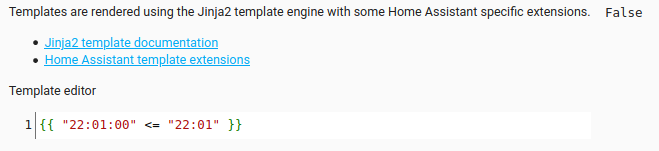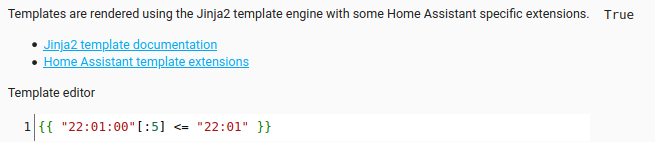# Need Help With Negative Values In Template - Weird Behaviour

Tags: #<Tag:0x00007f739aecd780>

I have a template which compares the solar angle to an input_number

``````{{ states('sensor.solar_angle') > states('input_number.hall_light_solar_angle_for_scene') }}
``````

If the solar angle is less than -2 and the input number is between -2 and 0, it gives a true result when I’d expect it to be false:

For example, the following template in the template editor produces the following result:

``````{{ states('sensor.solar_angle') > states('input_number.hall_light_solar_angle_for_scene') }}
{{ states('sensor.solar_angle')}}
{{ states('input_number.hall_light_solar_angle_for_scene') }}
``````

Result

``````True
-10.16
-1.9
``````

The result should be false as -10.16 is not greater than -1.9.

The solar angle sensor is taken from the sun attribute:

``````solar_angle:
friendly_name: "Sun angle"
unit_of_measurement: 'degrees'
value_template: "{{ state_attr('sun.sun', 'elevation') }}"
``````

Your template doesn’t use `int` or `float` to convert the output of the `states()` function to a numeric value. That means it’s comparing string values, as opposed to numeric values. That explains the results you see.

Use the `float` filter to convert each string value to a numeric and then the comparisons will work the way you expect.

``````{{ states('sensor.solar_angle') | float > states('input_number.hall_light_solar_angle_for_scene') | float }}
``````

Perfect, that works now!

Don’t suppose you know how I should compare timestamps (HH:MM format)

``````{{ states('input_datetime.landing_light_time_for_scene') <= states('sensor.time') }}
``````

The template above seems to work and not given me any issues, but you’ve got me thinking if i should covert the outputs here as well??

Don’t attempt to convert the values in that one. A string comparison happens to be acceptable in this very specific use-case where the two strings represent time.

Except that the input_datetime will return a string value including seconds.
As Taras says the string comparison will still evaluate correctly but it will be : -
‘22:01:00’ <= ‘22:02’ returns true but
What about ‘22:01:00’ <= ‘22:01’ ???
You would probably be fine and never notice if there was a minute difference but the OCD in me will instead use : -

``````{{ states('input_datetime.landing_light_time_for_scene') [0:5] <= states('sensor.time') }
``````

As that will compare ‘22:01’ <= '22:01

1 LikeMutt makes a valid point. Best to slice off the seconds in the input_datetime’s value (`HH:MM:SS`) when comparing to sensor.time’s value (`HH:MM`).